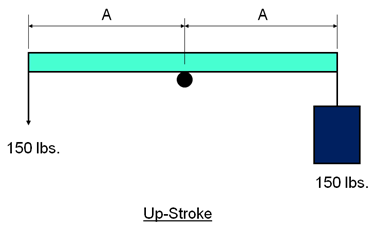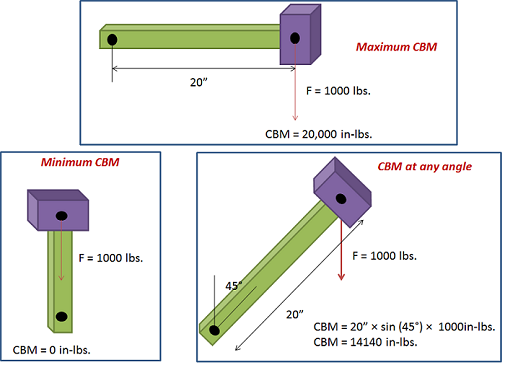## Why Do We Counterbalance Sucker Rod Pumping Units?

A good understanding of counterbalance is vital to the successful operation of surface sucker rod pumping units.  Poor counterbalance practices can cause early failure of the gear reducer gearing and will result in excessive energy cost.

### Non-counterbalanced lever system:

The up-stroke:The figure above represents a simple non-counterbalanced lever system. On the up-stroke, by pulling down on the end of a beam, a man is lifting a bucket full of water having a combined weight of 150 lbs.

Note that the upstroke effort of the man is a substantial 150 lbs.

## Torque and Maximum Counterbalance Moment

Torque is defined as twisting force. To calculate the torque around the rotation of a crank caused by a weight at the end of the crank, you need to multiply the weight times the horizontal distance from the center of gravity of the weight to point of rotation.

Unit of Torque:

The International System of Units, SI, (the French Système International (d’unités)), suggests using the unit newton meter (N⋅m). The unit newton meter is properly denoted N⋅m or N m. This avoids ambiguity with mN (millinewtons).

In Imperial units, “pound-force-feet” (lbf-ft), “foot-pounds-force”, “inch-pounds-force” are used. Other non-SI units of torque include “meter-kilograms-force” are also used. For all these units, the word “force” is often left out. For example, abbreviating “pound-force-foot” to simply “pound-foot” (in this case, it would be implicit that the “pound” is pound-force and not pound-mass).

### Maximum and Minimum Counterbalance Moment:

The crank generates maximum torque when it is in a horizontal position. This maximum torque is known as the Maximum Counterbalance Moment” ( maximum CBM) expressed in inch-pounds.NB: In rod pumping, the CBM is expressed in thousands of inch pounds.